# Fractal Art, Fractal Geometry & Fractal Patterns

Posted by Randall Morgan on

# Fractal Art, Fractal Geometry & Fractal Patterns## Why are fractal patterns interesting?

Fractals are interesting for many reasons. One interesting aspect of fractals is that they often exhibit self-similarity, meaning that the same pattern appears at different scales. This can result in visually striking and complex shapes that are created by repeating a simple process over and over. Another interesting aspect of fractals is that they can be found in a wide variety of natural phenomena, such as in the shapes of snowflakes, trees, and rivers. Finally, fractals have been used in a variety of fields, including mathematics, physics, and computer science, to model and understand complex systems.

## Types of Fractals

There are many different types of fractals, and they can be broadly classified into several categories. Some common categories of fractals include:

• Geometric fractals: These are fractals that are created using geometric shapes and repeating patterns. Examples include the Mandelbrot set and the Sierpinski triangle.
• Natural fractals: These are fractals that occur naturally in the world around us. Examples include the shapes of snowflakes, trees, and rivers.
• Iterated function system (IFS) fractals: These are fractals that are created using a set of simple rules that are repeated iteratively.
• Random fractals: These are fractals that are generated using random processes. An example is the Brownian tree.
• Fractal art: This is a category of art that is created using fractal patterns and techniques.
• Fractal music: This is music that is generated using fractal algorithms.

These are just a few examples, and there are many other types of fractals as well.

## How are fractals used in the real world?

Fractals have been used in a variety of ways in technology. Some examples include:

• Data compression: Fractal algorithms have been used to compress images, audio, and other types of data. Because fractals can represent complex patterns with a relatively small amount of information, they can be used to create highly efficient data compression schemes.
• Computer graphics: Fractals have been used to generate computer-generated images and animations. They can be used to create realistic landscapes, plant life, and other natural-looking objects.
• Image processing: Fractals have been used to process and analyze images in a variety of applications, including medical imaging, satellite imagery, and microscopy.
• Antenna design: Fractal shapes have been used to design antennas that are highly efficient at transmitting and receiving signals over a wide range of frequencies.
• Computer-aided design: Fractals have been used to create efficient algorithms for computer-aided design (CAD) applications, which are used to design everything from airplanes to electronic circuits.
• Data storage: Fractals have been used to design efficient data structures for storing large amounts of data in computers.

Overall, the use of fractals in technology has led to the development of a wide range of innovative applications and has had a significant impact on many fields.

## Fractal Meaning:

1. Mathematics, Physics. an irregular geometric structure that cannot be described by classical geometry because magnification of the structure reveals repeated patterns of similarly irregular, but progressively smaller, dimensions: fractals are especially apparent in natural forms and phenomena because the geometric properties of the physical world are largely abstract, as with clouds, crystals, tree bark, or the path of lightning.
2. Architecture, Decorative Art. a design or construction that uses the concept and mechanics of fractal geometry.

The English word "fractal" comes from Latin word "fractus", which means "fractured"

## Fractals in Nature: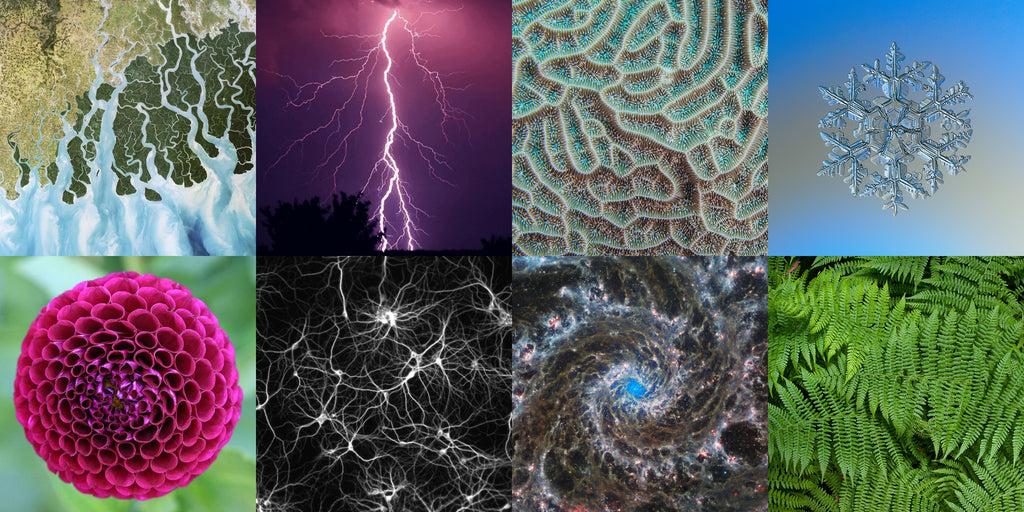Fractals can be found in a wide variety of natural phenomena and are used to model and understand complex systems in a variety of fields. For example, fractals have been used to model the shapes of coastlines, the structure of snowflakes, and the patterns found in plants and animals. In finance, fractals have been used to model market behavior and to identify patterns in stock prices. In medicine, fractals have been used to study the complexity of heart rhythms and to understand the structure of proteins.

In geology, fractals have been used to understand the patterns found in geological structures such as mountains and river networks. Additionally, fractals have been used in computer science to create efficient data structures and to design algorithms for image processing and other tasks. Overall, the study of fractals has led to a deeper understanding of many complex systems and has had numerous practical applications.

## Fractal Geometry

### Mandelbrot Fractal“Bottomless wonders spring from simple rules which are repeated without end.” – Benoît Mandelbröt  (1924-2010)

The Mandelbrot Set - Benoit Mandelbrot is often cited as the “father of fractal geometry” for the pioneering use of computers for research. In 1982 he released his book “The Fractal Geometry of Nature” altering the field of applied mathematics.

The Mandelbrot set is a mathematical object that is created by iterating a complex function over and over. The equation that is used to generate the Mandelbrot set is:

Z(n+1) = Z(n)^2 + C

where Z(n) is a complex number and C is a constant complex number.

The Mandelbrot set is created by starting with a value for C and iterating the equation above a large number of times. If the magnitude of Z(n) remains bounded as the equation is iterated, then the point C is considered to be a member of the Mandelbrot set. If the magnitude of Z(n) grows without bound as the equation is iterated, then the point C is not a member of the set.

The Mandelbrot set is plotted on the complex plane, with the real part of C on the x-axis and the imaginary part of C on the y-axis. The resulting plot is a highly intricate and complex pattern that exhibits self-similarity and has become one of the most well-known and studied fractals in mathematics.

### Julia Set FractalJulia sets are a type of fractal that is created using a similar process to the Mandelbrot set. Instead of using a constant value for C, Julia sets use a fixed value for Z(0) and vary the value of C to create the fractal.

The equation used to generate Julia sets is:

Z(n+1) = Z(n)^2 + C

where Z(n) is a complex number and C is a constant complex number.

As with the Mandelbrot set, the Julia set is plotted on the complex plane, with the real part of C on the x-axis and the imaginary part of C on the y-axis. The resulting plot is a highly intricate and complex pattern that exhibits self-similarity and can be used to study the behavior of iterated functions.

Julia sets can be used to study a wide range of mathematical and scientific phenomena, and are an important tool in the field of complex dynamics.

### Phoenix Fractal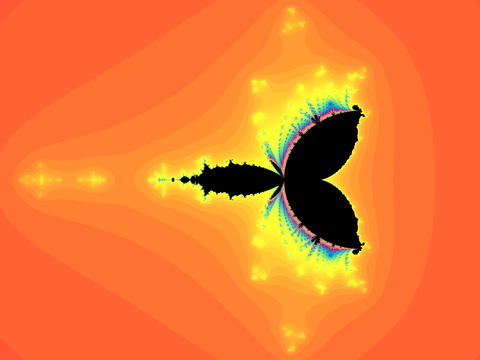The Phoenix fractal is a modification of the Mandelbrot and Julia sets. It was discovered by Shigehiro Ushiki in 1998 in a paper published in the 'IEEE Transactions on Circuits and Systems' journal.

### Newton FractalThe Newton fractal is a boundary set in the complex plane. The fractal is characterized by Newton's method (a well known procedure for finding roots of functions) applied to a fixed transcendental function.

Most people learn Newton's method using real functions, however it can be applied to complex functions, such as in the Newton Fractal.

### Koch Fractal (Koch Snowflake)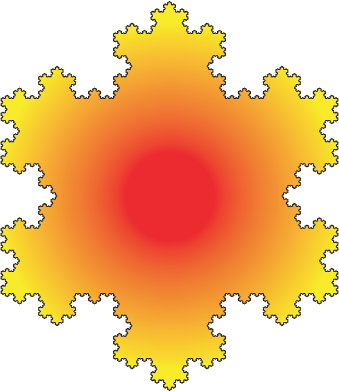The Koch Snowflake fractal (also known as Koch curve or Koch star) is a fractal curve and one of the earliest known fractals to have been discovered. It was first described in 1904 by a Swedish mathematician Helge von Koch.

The Koch Snowflake can be built up iteratively. The above illustration is a Koch snowflake with 5 iterations. Iteration 1 is a simple equilateral triangle. This iterative process can go on forever, creating an infinite fractal curve. This creates a fractal that encloses a finite area, but has an infinite perimeter.

### Fibonacci FractalIn geometry the golden spiral is a type of logarithmic spiral whose growth factor is the golden ratio. The Fibonacci spiral is an approximation of the golden spiral and gets closer as the numbers increase toward infinity.

Approximations of logarithmic spirals can occur in nature at various scales. A prime example being spiral galaxies.

### Other types of fractals include:

####The Barnsley fern is a fractal named after British mathematician Michael Barnsley, who first described it in his book 'Fractals Everywhere'.  The book was based on courses Barnsley taught at the Georgia Institute of Technology.  This is an example of using an iterated function system (IFS) to develop a fractal.

#### Sierpinski Carpet Fractal: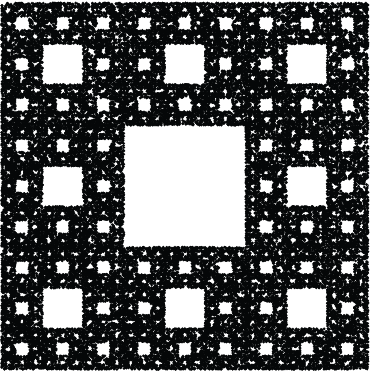The Sierpinski carpet is a fractal that was first described in 1916 by a Polish mathematician named Wacław Sierpiński. The same method used to create the Sierpinski carpet also works with other shapes such as the equilateral triangle or a pentagon.

#### Sierpinski Triangle Fractal:#### Sierpinski Pentagon Fractal:#### Harter-Heighway Dragon Fractal: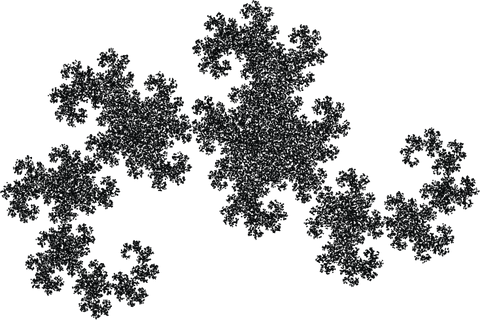The Heighway dragon fractal was first investigated by NASA physicist John Heighway. Details of this fractal were first published in 1957 in the Scientific American magazine.

## Fractal Art

### What makes fractal art so unique?

Fractal art is unique in several ways. One key aspect of fractal art is that it is created using mathematical algorithms that generate intricate and complex patterns. These patterns can be highly detailed and can exhibit self-similarity, meaning that the same pattern appears at different scales. Fractal art can also be highly colorful and can incorporate a wide range of color schemes and palettes.

Another unique aspect of fractal art is that it is created using computers, which allows for the rapid exploration and experimentation with different algorithms and parameters. This makes it possible for artists to create a wide range of different styles and looks, and to easily fine-tune and adjust their artwork.

Finally, fractal art is often associated with a sense of mystery and otherworldliness, as it often creates patterns and shapes that do not occur in nature. This can give fractal art a unique and otherworldly feel that sets it apart from other types of art.

### Fractal Artist: Pardesco

Pardesco is a well-known fractal artist who has been creating detailed fractal carvings since 2020. Using advanced 3D CNC machining techniques, Pardesco is able to create intricate and highly detailed fractal patterns in wood, bringing these mathematical patterns to life in a tactile and sculptural form. Pardesco's fractal carvings are known for their incredible level of detail and the way they seem to almost shimmer and shift as the viewer moves around them. The use of 3D CNC machining allows Pardesco to precisely control the depth and shape of the carvings, adding another level of detail and complexity to the work. Whether viewed up close or from a distance, Pardesco's fractal carvings are a testament to the beauty and complexity of these mathematical patterns and are sure to captivate and inspire viewers of all ages.

## Fractal Patterns

Thank you for visiting our fractal blog! We hope you enjoyed learning about these fascinating mathematical patterns and their many applications. As a token of our appreciation, we would like to offer you a free gift of four high-resolution 4K desktop wallpapers featuring stunning fractal patterns. Simply click on the link below to download these wallpapers and use them to add a touch of fractal beauty to your desktop. Thank you again for visiting and we hope to see you back on our blog soon!

###### Preview of colorful fractal wallpapers 4kPreview of dark fractal wallpapers 4k### A poem about the Mandelbrot set

prompted by Pardesco using AI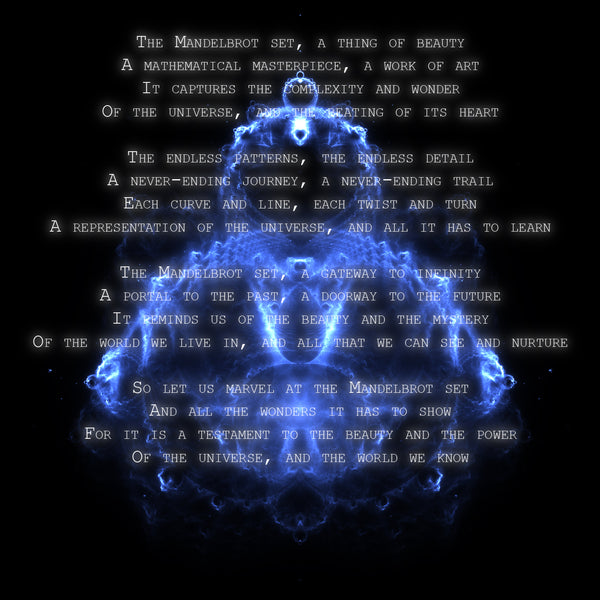Thank you for taking the time to read our blog. If you enjoyed the content and want to support the artist, please consider purchasing some of their artwork.

Your support helps to sustain their work and allows them to continue creating beautiful and meaningful pieces for you to enjoy. Thank you for your consideration and we hope to see you again soon!